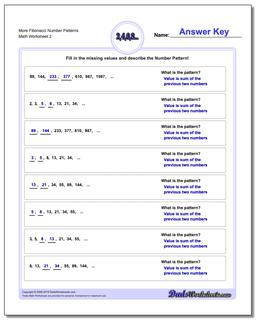PLEASE GO BACK AND USE THE BIG BLUE 'PRINT' BUTTON ON THE PAGE TO PRINT THE WORKSHEET CORRECTLY!Sorry for the trouble! The browser won't print the embedded worksheet PDF directly using the normal 'Print' command in the file menu, so you need to click the big 'Print' button to send just the worksheet and not the surrounding page to the printer.

# Math Worksheets: Number Patterns: Number Patterns: More Fibonacci Number Patterns (Second Worksheet)## More Fibonacci Number Patterns (Second Worksheet)

PropertyValue
DescriptionMore Fibonacci Number Patterns: Fibonacci and Fibonacci-like number pattern problems, where the next values in a sequence are dependent on prior values. (Second Worksheet)
Resource TypeWorksheet